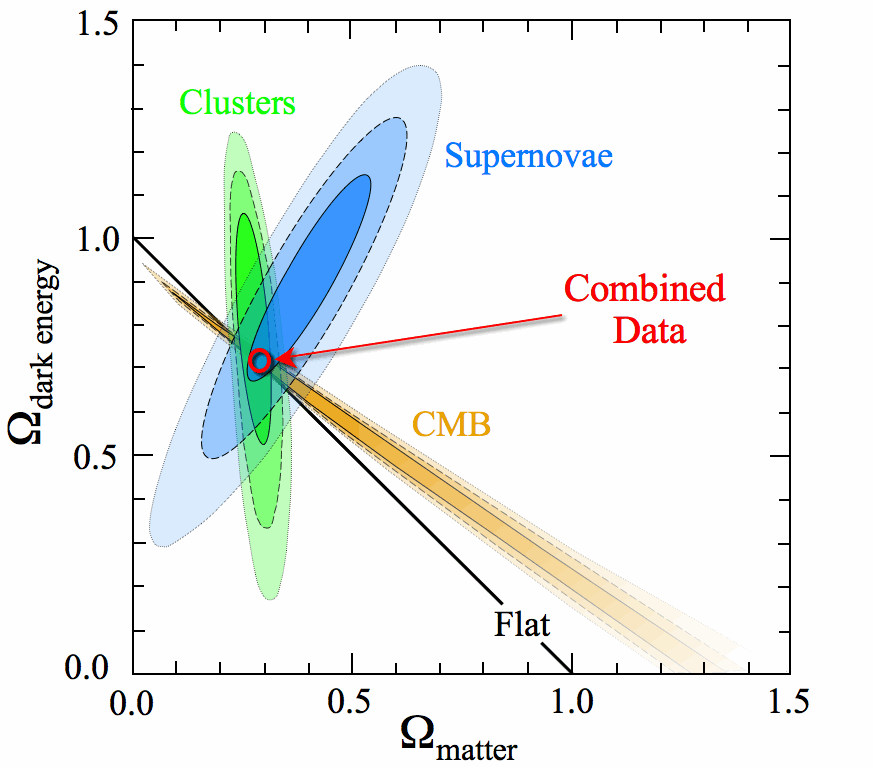Contents

Idea

The standard model (in theoretical physics) for the observable universe on the largest length scales of cosmology:

it is an inflationary FRW spacetime with cosmological constant (“dark energy”) and cold dark matter. The technical term for this is the $\Lambda$ CDM concordance model (where “$\Lambda$” is the standard symbol for the cosmological constant and “CDM” is for “cold dark matter”).

The current model assumes that the energy density of the observable universe consists of(e.g. Einasto 09, fig 17, here)

Properties

Structure formation

Computer simulation of cosmic structure formation on scales larger than that of galaxies had always shown very good agreement of the $\Lambda CDM$ standard model of cosmology and observation.

There used to be various discrepancies of cold dark matter-models on the scale of galaxies

But recent analysis seems to show that more fine-grained analysis shows that cold dark matter-models match all of these observations well. See behind the above links for more.

Vacuum energy and Cosmological constant

The renormalization freedom in perturbative quantization of gravity (perturbative quantum gravity) induces freedom in the choice of vacuum expectation value of the stress-energy tensor and hence in the cosmological constant.

For review see Hack 15, section 3.2.1

For more see at cosmological constant here.

standard model of particle physics and cosmology

theory:Einstein-Yang-Mills-Dirac-Higgs
gravityelectroweak and strong nuclear forcefermionic matterscalar field
field content:vielbein field $e$principal connection $\nabla$spinor $\psi$scalar field $H$
Lagrangian:scalar curvature densityfield strength squaredDirac operator component densityfield strength squared + potential density
$L =$$R(e) vol(e) +$$\langle F_\nabla \wedge \star_e F_\nabla\rangle +$$(\psi , D_{(e,\nabla)} \psi) vol(e) +$$\nabla \bar H \wedge \star_e \nabla H + \left(\lambda {\vert H\vert}^4 - \mu^2 {\vert H\vert}^2 \right) vol(e)$

General

Lecture notes include

Review:

In March 2013, following an accurate processing of available measurement data, the Planck Scientific Collaboration published the highest-resolution photograph ever of the early Universe when it was only a few hundred thousand years old. The photograph showed galactic seeds in sufficient detail to test some nontrivial theoretical predictions made more than thirty years ago. Most amazing was that all predictions were confirmed to be remarkably accurate. With no exaggeration, we may consider it established experimentally that quantum physics, which is normally assumed to be relevant on the atomic and subatomic scale, also works on the scale of the entire Universe, determining its structure with all its galaxies, stars, and planets.

• George Efstathiou, Steven Gratton, The evidence for a spatially flat Universe (arXiv:2002.06892)

A discussion of open problems is in

Possible tension with observation

Possible tensions in the model, regarding determination of the Hubble constant in the early and late universe, apparently observed by various groups, since the late 2010s:

review:

• L. Verde T. Treu, A.G. Riess, Tensions between the Early and the Late Universe (arXiv:1907.10625)

Indication that the tension is not in the data but in systematic errors:

• Freedman et al. The Carnegie-Chicago Hubble Program. VIII. An Independent Determination of the Hubble Constant Based on the Tip of the Red Giant Branch (arxiv:1907.05922)

In AQFT on Curved spacetimes

Discussion in the rigorous context ofAQFT on curved spacetimes includes

For review see